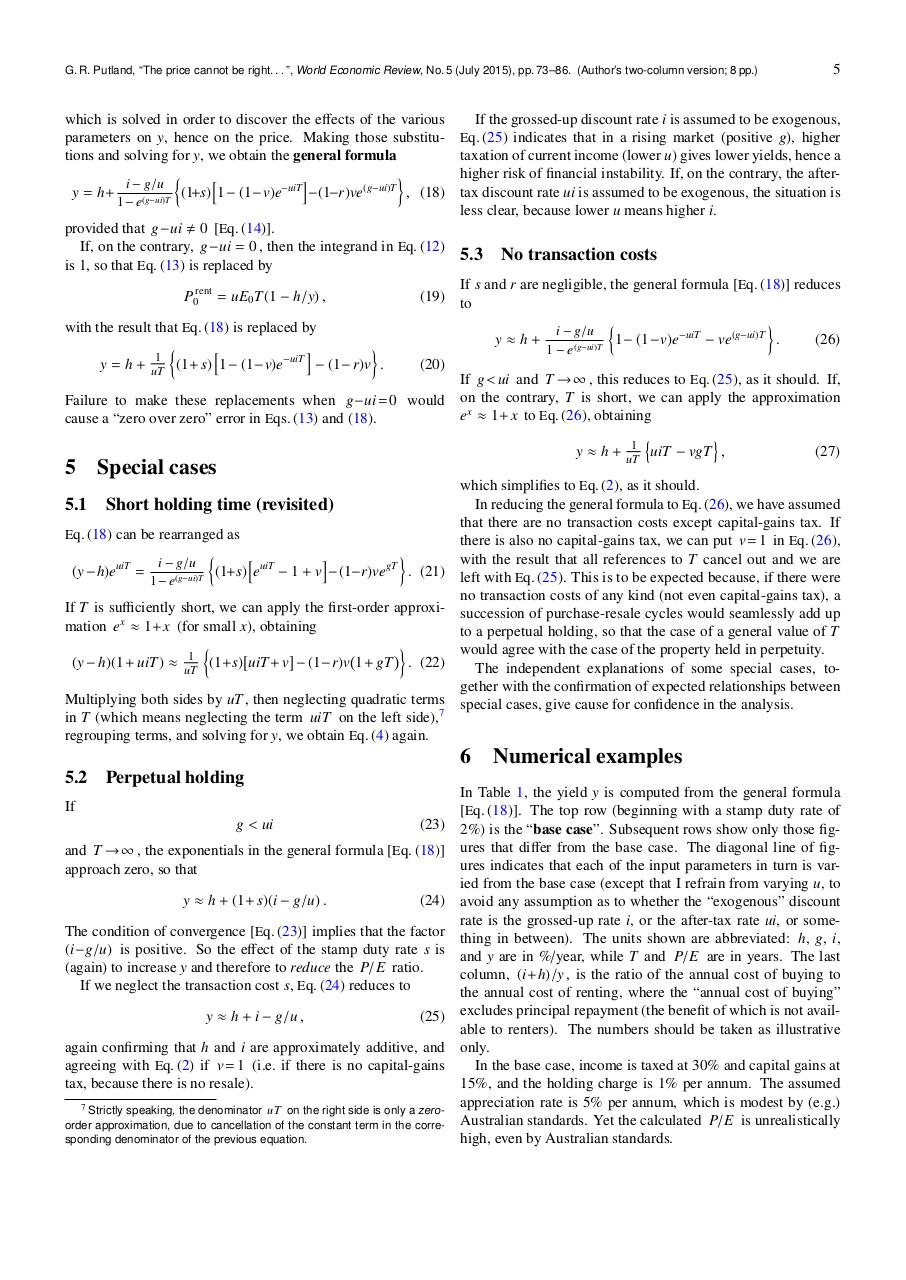# PDF Archive

Easily share your PDF documents with your contacts, on the Web and Social Networks.

## putland wer 5 2col.pdfPage 1 2 3 4 5 6 7 8

#### Text preview

G. R. Putland, “The price cannot be right. . . ”, World Economic Review, No. 5 (July 2015), pp. 73–86. (Author’s two-column version; 8 pp.)

which is solved in order to discover the effects of the various
parameters on y, hence on the price. Making those substitutions and solving for y, we obtain the general formula

h
i
i − g/u
−uiT
(g−ui)T
, (18)
(1+s)
1−
(1−v)e
−(1−r)ve
y = h+
(g−ui)T
1− e

provided that g−ui , 0 [Eq. (14)].
If, on the contrary, g−ui = 0 , then the integrand in Eq. (12)
is 1, so that Eq. (13) is replaced by
P0rent = uE0 T (1 − h/y) ,
with the result that Eq. (18) is replaced by

h
i
1
y = h + uT
(1+ s) 1− (1−v)e−uiT − (1− r)v .

If the grossed-up discount rate i is assumed to be exogenous,
Eq. (25) indicates that in a rising market (positive g), higher

taxation of current income (lower u) gives lower yields, hence a
higher risk of financial instability. If, on the contrary, the aftertax discount rate ui is assumed to be exogenous, the situation is
less clear, because lower u means higher i.

5.3

No transaction costs

If s and r are negligible, the general formula [Eq. (18)] reduces
(19) to

i − g/u
−uiT
(g−ui)T
.
(26)
1−
(1−v)e

ve
y≈h+
(g−ui)T
1−e

(20)

Failure to make these replacements when g−ui = 0 would
cause a “zero over zero” error in Eqs. (13) and (18).

5

5

Special cases

If g &lt; ui and T → ∞ , this reduces to Eq. (25), as it should. If,
on the contrary, T is short, we can apply the approximation
e x ≈ 1+ x to Eq. (26), obtaining
n
o
1
y ≈ h + uT
uiT − vgT ,
(27)

which simplifies to Eq. (2), as it should.
In reducing the general formula to Eq. (26), we have assumed
that there are no transaction costs except capital-gains tax. If
Eq. (18) can be rearranged as
there is also no capital-gains tax, we can put v = 1 in Eq. (26),

h
i
with the result that all references to T cancel out and we are
i − g/u
(1+s) euiT − 1 + v − (1−r)vegT . (21) left with Eq. (25). This is to be expected because, if there were
(y − h)euiT =
(g−ui)T
1− e
no transaction costs of any kind (not even capital-gains tax), a
If T is sufficiently short, we can apply the first-order approxi- succession of purchase-resale cycles would seamlessly add up
mation e x ≈ 1+ x (for small x), obtaining
to a perpetual holding, so that the case of a general value of T

would agree with the case of the property held in perpetuity.

1
(y − h)(1+ uiT ) ≈ uT
(1+s) uiT + v − (1−r)v 1+ gT . (22)
The independent explanations of some special cases, together with the confirmation of expected relationships between
Multiplying both sides by uT , then neglecting quadratic terms special cases, give cause for confidence in the analysis.
in T (which means neglecting the term uiT on the left side),7
regrouping terms, and solving for y, we obtain Eq. (4) again.

5.1

Short holding time (revisited)

5.2

Perpetual holding

6
If
g &lt; ui

(23)

and T → ∞ , the exponentials in the general formula [Eq. (18)]
approach zero, so that
y ≈ h + (1+ s)(i − g/u) .

(24)

The condition of convergence [Eq. (23)] implies that the factor
(i−g/u) is positive. So the effect of the stamp duty rate s is
(again) to increase y and therefore to reduce the P/E ratio.
If we neglect the transaction cost s, Eq. (24) reduces to
y ≈ h + i − g/u ,

(25)

again confirming that h and i are approximately additive, and
agreeing with Eq. (2) if v = 1 (i.e. if there is no capital-gains
tax, because there is no resale).
7 Strictly speaking, the denominator uT on the right side is only a zeroorder approximation, due to cancellation of the constant term in the corresponding denominator of the previous equation.

Numerical examples

In Table 1, the yield y is computed from the general formula
[Eq. (18)]. The top row (beginning with a stamp duty rate of
2%) is the “base case”. Subsequent rows show only those figures that differ from the base case. The diagonal line of figures indicates that each of the input parameters in turn is varied from the base case (except that I refrain from varying u, to
avoid any assumption as to whether the “exogenous” discount
rate is the grossed-up rate i, or the after-tax rate ui, or something in between). The units shown are abbreviated: h, g, i,
and y are in %/year, while T and P/E are in years. The last
column, (i+h)/y , is the ratio of the annual cost of buying to
the annual cost of renting, where the “annual cost of buying”
excludes principal repayment (the benefit of which is not available to renters). The numbers should be taken as illustrative
only.
In the base case, income is taxed at 30% and capital gains at
15%, and the holding charge is 1% per annum. The assumed
appreciation rate is 5% per annum, which is modest by (e.g.)
Australian standards. Yet the calculated P/E is unrealistically
high, even by Australian standards.